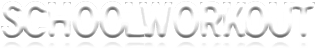Numeracy

This web page contains teaching resources for Numeracy.  There are PowerPoints, games and worksheets that can be downloaded.

 primary pages for teachers numeracy literacy science history geography other subjects home

General resources

A useful booklist from the DfES of maths through stories.

Year 1

The files below can be downloaded - they contain resources suitable for Year 1 Numeracy lessons.

• PowerPoint presentation covering addition and subtraction problems - numbers to 20

Year 4

The files below contain resources suitable for Year 4 Numeracy lessons.

• Maths Times Table Challenge (3 times and 4 times tables)

• Maths Times Table Challenge (6 times and 7 times tables)

Year 6

The files below contain resources suitable for Year 6 Numeracy lessons.

NUMBER

• Matching game - match percentages to shaded fractions of a square

• Matching game - match percentages to fractions

MEASURES

• Follow-me game - conversions between metric units of length

• Worksheet - converting between metric units of length

• Worksheet - equivalence between metric and imperial units

SHAPE AND SPACE

• Sorting activity - side and angle properties of different types of quadrilaterals

• Worksheet - diagonal properties of quadrilaterals

HANDLING DATA

• Worksheet - shading spinners to match probability statements (sheet 1)

• Worksheet - shading spinners to match probability statements (sheet 2)

• Activity - sorting probability statements